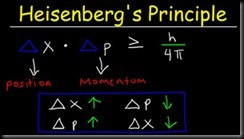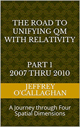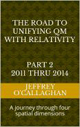Quantum mechanics states what the universe is made of while not giving an explanation of why it is that way while Relativity gives us an explanation of why it is what it is but does not tell us what is it made of.  For example, a quantum world is defined in terms of the wave-particle duality of existence while its interactions with that world are defined in terms probabilities and the uncertainty principal which states one cannot precisely measure the properties of Conjugate pairs such as the momentum or position of a particle with complete accuracy.  However, it does not give an explanation of what existence is or how it interacts with its environment to create the universe we live in.  On the other hand, Relativity explains the existence of the universe in terms of an interaction between space and time without telling us what wave-particle duality of existence is or how it interacts with it to create the uncertainty principal.However, to understand the dynamics of the uncertainty principle in terms of space-time we must first establish a physical connection between the wave function and the properties of the space-time. This can be accomplished because in Relativity the evolution of space-time is defined in terms of an electromagnetic wave while, as was mentioned earlier the wave function represents how the quantum world evolves to the point where it is observed.

This commonality suggests the wave function could be represented by an electromagnetic wave in space-time.  This means to derive the wave particle duality of existence defined by the wave function in terms of space-time one must physically connect the evolution of an electromagnetic wave to its existence.

This is possible because the science of wave mechanics and Relatively tells us an electromagnetic wave moves continuously through space-time unless it is prevented from moving through time by someone or something interacting with it. This would result in it being confined to three-dimensional space. The science of wave mechanics also tells us the three-dimensional "walls" of this confinement will result in its energy being reflected back on itself thereby creating a resonant or standing wave in three-dimensional space. This would cause the energy of an electromagnetic wave to be concentrated at the point in space were a particle would be found.  Additionally, wave mechanics also tells us the energy of a resonant system such as a standing wave can only take on the discrete or quantized values associated with its fundamental or a harmonic of its fundamental frequency.

For example, it explains why if the wave function is prevented from evolving through the probability field that defines a quantum existence by an observation it presents itself as a particle in terms of the properties of an electromagnetic wave in a space-time environment.

Yet, it also tells us, similar to the evolution of an electromagnetic wave in space-time if unobserved the it will continue evolve through the probabilistic universe defined by quantum mechanics.

In other words, it shows how one can understand the evolution of wave-particle duality of a quantum existence by comparing it to the evolution of an electromagnetic wave in space-time

Next, we must explain how quantum mechanics definition of a particle in terms of a one-dimensional point is responsible for the validity of the uncertainty principal.

Relativity and the science of wave mechanics tell us the energy of the standing wave which earlier defined a particle would be distributed over a volume of space-time that corresponds to is wavelength. However, to accurately determine its momentum or position one must be able to determine where those measurement are taken with respect to energy volume those system occupy.

Yet, to measure momentum of a particle in the quantum world one must determine time it takes to move between two points in the probability field.  Therefore, they will be an inherent uncertainty if one cannot determine where with respect to volume of the system those points are.

But because in Relativity the measurement of position is taken with respect to its spatial not its time properties one should define it with respect to space not time

Einstein gave us the ability to do this when he defined the mathematical relationship between space and time in terms of the constant velocity of light because in doing so, he provided a method of converting a unit of time in a space-time environment to its equivalent unit of space in four *spatial* dimensions.  Additionally, because the velocity of light is constant, he also defined a one to one quantitative and qualitative correspondence between his space-time universe and one made up of only four *spatial* dimensions.

In other words, one can use a displacement in the "surface" of three-dimensional space with respect to a fourth *spatial* dimension to define a particles position while using a displacement with respect to a time dimension to define its momentum.

As was mentioned earlier Einstein defined the energy volume of a system in terms of a displacement in space-time and because energy can neither be created or destroyed it will remain constant therefore the volume of the displacement in space time will also remain constant.

HOWEVER, BECAUSE QUANTUM MECHANICS IS INFORMATION BASED ONE CAN SAY THE INFORMATION VOLUME OF A SYSTEM ALSO REMAINS CONSTANT.

Therefore, because the measurement of monument or position does not change the energy of a system the combined information volume associated with momentum and the displacement associated with position remains constant.

As was also mentioned earlier Quantum mechanics defines both moment and position with respect to a one-dimensional point in a probability field.  However, the accuracy of the information as to where that point is in relation to its information volume is directly related to how much information is taken from the system.  In other words, the more accurate the measurement the more information regarding it must be removed from the system.

However, because, as was mentioned earlier the information volume of a system remains constant the more information taken out of it regarding its momentum will result in there being more space for the point defining its position to occupy. This makes the determination of its position more uncertain because it could be found in anywhere in that larger volume.

This shows how one can define a dynamic relationship between the uncertainty of determining the momentum or position of a particle in terms of interaction between space and time and why it is responsible for the uncertainty principal.  This is because it defines why in terms of the properties of space-time the more information you have about the momentum of a particle the less you can know about it position and the more information you have about its position the less you can know about its momentum.

In other words, it shows how a dynamic interaction between the spatial and time properties of the universe can not only explain the particle wave duality of existence but also the uncertainty principal of quantum mechanics

Copyright Jeffery B O’Callaghan Nov. 2020

The Road to unification part  2007 thru 2010  Ebook

 The Road to Unification part 1 2007 thru 2010Ebook \$8.00Paper Back \$15.00 The Road to Unification part 2 2011 thru 2014Ebook \$8.00Paper Back \$17.00 The Road to Unification part 3 2015 thru 2020Ebook \$8.00Paper Back \$15.00

Unifying Quantum and Relativistic Theories is based on WordPress platform.